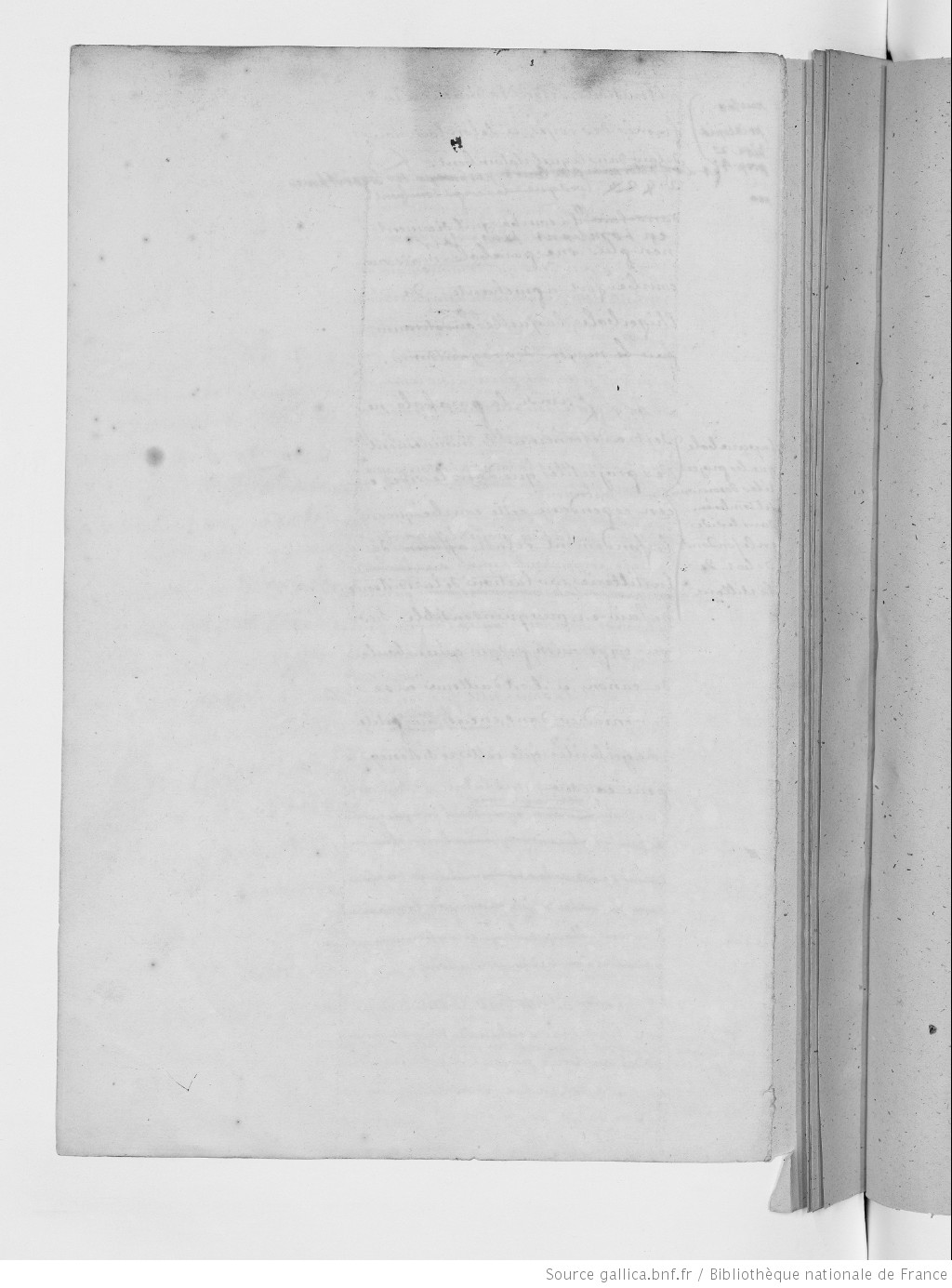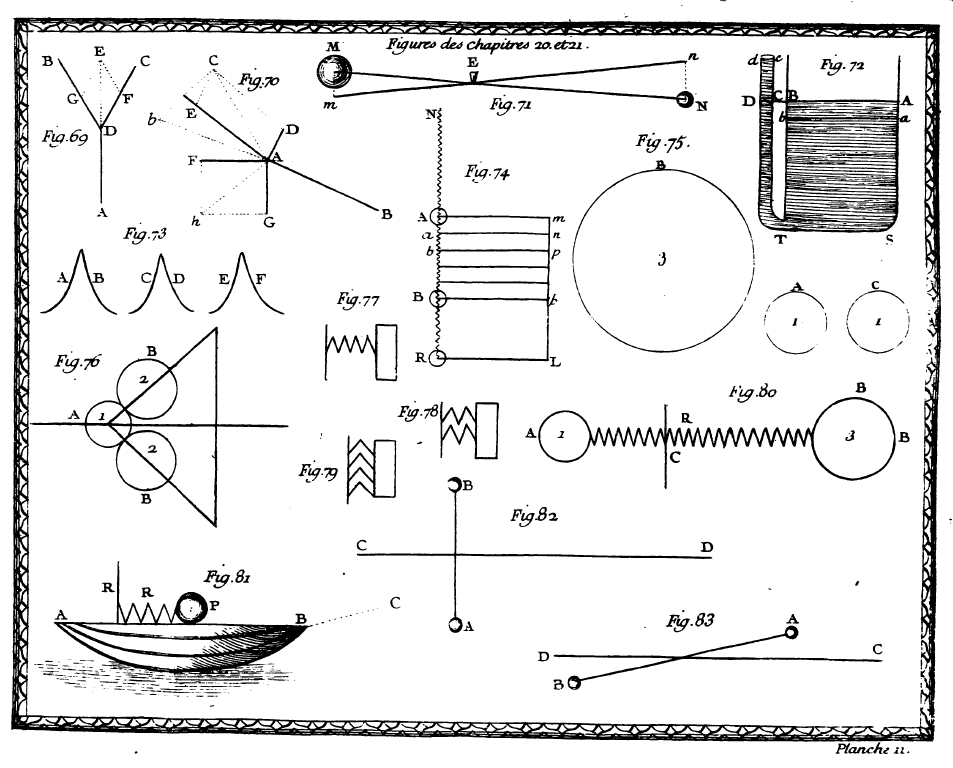Chapter 20. Of Dead Forces, or Pressing Forces, and the Equilibrium of Powers

At the beginning of Chapter 20, Du Châtelet distinguishes between two kinds of moving, or motive forces  (InstPhy, § 519):

One can distinguish two kinds of moving forces: dead force and living force. Dead force consists in a simple tendency to motion: for example, the tendency of a spring when it is ready to uncoil. When a body it is actually in motion it has a living force. (Copyright © 2018 KB)

Given this distinction, the crucial question is how living force can arise from dead force. Du Châtelet assumes that living force arises from dead force when a series of infinitely small forces or pressures act on a body. Both, dead forces and living forces, can be considered as either active or passive. Dead force produces no real effect, but only tends, at each instant, to produce one. The force that produces it is never exhausted, i.e. its effect never survives its action (InstPhy, §§ 528):

Although dead forces produce no effects, they can nevertheless be considered as either active or passive. The Dead Force that I consider as active is the force that bodies have by which to keep a power in equilibrium. The Dead Force that I consider as passive is that which a motionless body receives when it is solicited to move but nevertheless remains at rest. (Copyright © 2018 KB)

According to Du Châtelet, dead force and living force differ essentially from one another, since the former does not produce any real effect, and the latter does. They are heterogeneous quantities. Nevertheless, they are comparable by means of the infinitesimal calculus, which allows to preserve the differences between the things compared (InstPhy, § 535):

It can already be seen that dead force and living force differ essentially from one another, since the former does not produce any effect, and the latter produces a real effect, which is the displacement of the obstacle. Thus, the two kinds of force are heterogeneous quantities, and they differ infinitely. (Copyright © 2018 KB)

Dead forces are merely solicited to move without actually moving; living forces arise, according to Du Châtelet, from an infinite number of continuous impressions of dead forces (InstPhy, § 534):

When the obstacles upon which motive force acts are not invincible, the action of this force upon these obstacles is to make them leave their place; and then the small degrees of motion that this force communicates, at each infinitely small instant, to the body upon which it acts, accumulate and are conserved therein, and this force compels the body to change place: in this case dead force changes into living force. (Copyright © 2018 KB)In chapter 21, Du Châtelet explains in detail the differential method of deriving the living force from the dead force. This is the reason why in § 535 of the Amsterdam edition 1742 the analogy between the relation of dead to living force and the relation of a ligne to a surface is missing (InstPhy, § 535):

I will discuss living forces in Chapter 21. Here I examine only the effects of simple pressure. (Copyright © 2018 KB)

Du Châtelet defines and explains, what the intensity and equilibrium of powers means (InstPhy, § 539):

Powers can differ from one another with respect to the size of the masses they can transport, and with respect to the infinitely small space they can traverse with the transported masses in equal times. (Copyright © 2018 KB)

At first glance, this comes very closely to our modern definition of intensity which is the power transferred per unit area, where the area is measured on the plane perpendicular to the direction of propagation of the energy. However, methods and concepts are different. As understood today, the power is the rate of doing work or transferring heat, the amount of energy transferred or converted per unit time. At Du Châtelet’s time, however, a clear distinction between power and energy was not available. Using the geometrical method of ratios and proportions, Du Châtelet determines the equilibirum of the intensity of powers as follows (InstPhy, § 554, Fig. 70):

Let this point A be pulled by the five powers D, E, F, G, B so that the power B is in equilibrium with the four other powers D, E, F, G. If these five powers are respectively proportional to the lines AD, AE, AF, AG, AB then, once we have formed the triangle ADC or the parallelogram ADCE, powers AE and AD will be contained in the single power AC that acts in direction AC; thus powers AD, AE, and AC will be in equilibrium (as shown in the preceding section). (Copyright © 2018 KB)

Form today’s perspective, the equilibrium means that the net force acting on a body is zero. More generally, an equilibrium is established at a point where the gradient of potential energy is zero. Du Châtelet’s definition branches rudimentary approaches to this understanding. However, she confuses action and resistance. A balance, for example, is in equilibrium if the moment of the applied force is equal and opposite to the moment of resistance. Thus, it is not correct to derive their equality from the Third Law which assumes that action and reaction act on different bodies (not on one and the same).

##### Back to main project | Next chapter